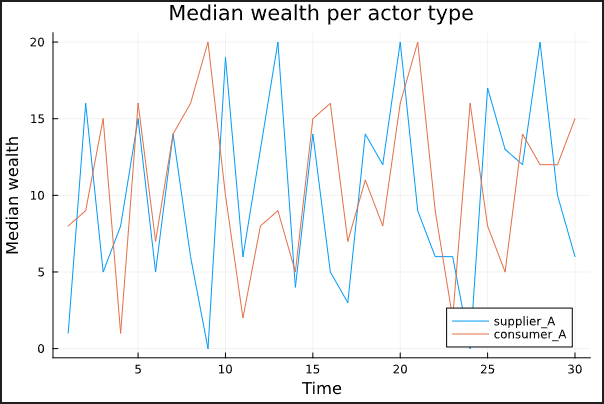# Trying to pass a variable to @df df plot(variable,

I’m trying to get the following code working but can’t figure out what I need to do.

``````function plot_type_wealth(dataframe)
type_vector = [:Supplier, :Consumer]
label_vector = ["Supplier" "Consumer"]

@df dataframe plot(type_vector,
title="Median wealth per actor type\n",
label=label_vector,
xlabel="Time",
ylabel="Median wealth")
end
``````

The following piece of code DOES work:

``````function plot_type_wealth(dataframe)
label_vector = ["Supplier" "Consumer"]

@df dataframe plot([:Supplier, :Consumer],
title="Median wealth per actor type\n",
label=label_vector,
xlabel="Time",
ylabel="Median wealth")
end
``````

The reason I want the first version to work is that I want to pass the columns that need to be plotted as a variable in the function. I know that it has to do with the @df macro but I can’t figure out how to also make it work with the variable.

Stef

I think I would just abandon the use of the macro in this case. Does this do what you want?

``````using DataFrames
using StatsPlots

df = DataFrame(
supplier_A=rand(0:20, 30),
consumer_A=rand(0:20, 30),
supplier_B=rand(0:20, 30),
consumer_B=rand(0:20,30)
)

function plot_type_wealth(df, cols)
plot(
[df[!, col] for col in cols],
label=reshape([string(col) for col in cols], 1, length(cols)),
title="Median wealth per actor type",
xlabel="Time",
ylabel="Median wealth"
)
end

plot_type_wealth(df, [:supplier_A, :consumer_A])
``````1 Like

You can’t make this work - the macro gets expanded before runtime and has no access to the value of `type_vector`.

What Matt says is right, if you want to use information on values do that with a regular function, not a macro.

2 Likes

Yes! That does the trick.

Thank you!